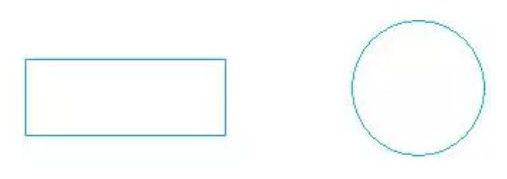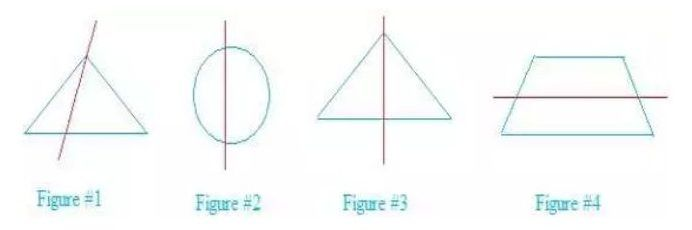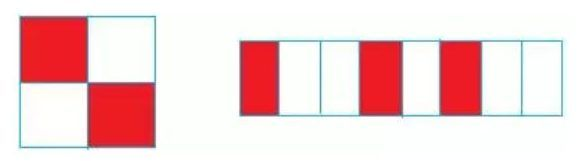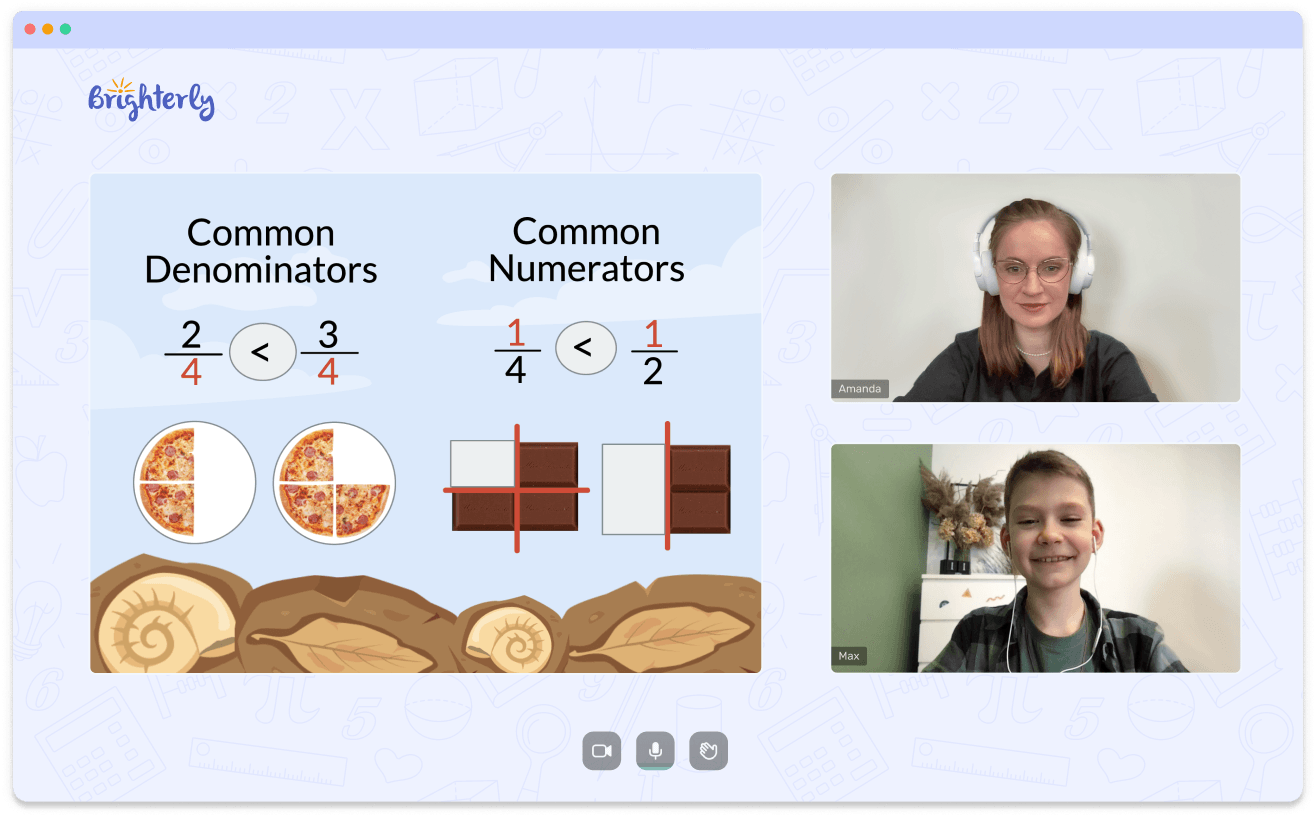# 2nd Grade Diagnostic Math Test

Math TestsAll second grade students will take 2 grade math tests irrespective of what type of education they’re receiving. Preparation for this math test for 2nd grade is an essential part of learning that can help kids advance.

The tests help kids interpret key numerical ideas, and teachers can use the results to pinpoint the areas where students might need extra help and direction. This article will go into details about these tests and provide a free sample for teachers to test their students.

## The Importance of the Diagnostic Test

Diagnostic tests are an essential part of education because they help teachers understand their students’ level and find areas where they can improve. By evaluating an understudy’s ongoing abilities, educators can adjust their approach to meet the particular requirements of every kid instead of expecting that all their students are at a similar level.

A student’s progress over time can be tracked with the help of a diagnostic second grade math test. Teachers can determine whether a student is progressing or struggling with a particular concept by administering the same test regularly. The information received is used to modify the teaching methods or provide additional assistance.

Additionally, diagnostic tests can assist students in comprehending their capabilities and limitations. Students can set goals for themselves and work toward improving their skills by identifying weaknesses. A student’s self-awareness can help them feel more confident and motivated to do well in school.

In general, 2nd grade diagnostic math tests are a helpful tool for educators, students, and parents to ensure students get the help they need to succeed in school.

A second grade math test typically covers various math concepts, such as essential addition and subtraction, place value, measurement, and geometry. To prepare for the test, students should review the math topics they have studied and practice solving problems that involve the ideas mentioned earlier. Students can also benefit from becoming familiar with the test’s format that may include multiple-choice or fill-in-the-blank questions.

Below is a 2nd grade math assessment test printable your kids can work on.

Print your second grade math test before you begin working on it. Try to respond to each question.

Find a solution to the following problems:

1. Which numbers come next?

16, 22, 28, _____, ____

2. Which coin is the largest and is worth less than fifty cents?

1. Dime
2. Quarter
3. Penny
4. Nickel
3. Divide the rectangle into three equally sized pieces and color one-third (or 1/3) of each. Divide the circle into four sections of equal size and color three-fourths (or 3/4) of it.4. Which one of these options is equivalent to two days?
1. Two weeks
2. Twenty-four hours
3. Twenty-four minutes
4. 2880 minutes
5. Please fill in the two omitted numbers

847, 855, ____, 871 , ____

6. Five pennies, three dimes, two nickels, and five quarters are in your pocket.

What amount of money do you have? How much money are you left with if you buy a candy for 65 cents?

1. \$1.65 plus the remaining \$1.00
2. \$1.70 plus the remaining \$1.00
3. \$1.70 plus the remaining \$1.05
4. \$1.65 plus the remaining \$1.05
7. At a wedding party, there are 458 visitors; however, only 324 dinners are served. How many additional meals are required?
8. Which figure is similar on the two sides of the red line?1. Figure 1
2. Figure 2
3. Figure 3
4. Figure 4
9. Determine whether the left number is lower or higher than the right number. Supplant the question mark ( ? ) with the “greater than” (>) or “less then” (<) sign

87         ?     81

157       ?     211

10. Which number has 2 in the position of ones and 5 in the hundreds?
1. 152
2. 252
3. 521
4. 512
11. What digit is in the tens, hundreds, and ones place in the number 985?

One__________ Ten____________, Hundred __________?

12. Solve the following adding and subtracting equations
13. Write the fractions in the space provided below the figures:14. Determine whether the left number is lower or higher than the right number. The question mark (?) should be replaced with <, =, or >

42 + 14?     56

65 + 33?     90

15. Put the following numbers in words:

25 ___________________ 48 ____________ 124 ___________________ 256 ____________

16. What is the name of a geometric figure that looks like the earth? Are Indian tents similar to tipis? Water-carrying pipe?
17. Please fill in the spaces for the missing numbers 467, 459, ______________, 443, 435____________
18. What is the length in red below measured in?

8th Grade Math Test – Diagnostic & Assessment Test for Eighth Grade

19. Which mathematical problem has the same answer as 20 + 8 = 28?
1. 20 − 8 = 12
2. 28 − 8 = 20
3. 28 − 8 = 18
4. 8 − 28 = 20
20. Math challenges: What am I?

a) 55 + 66 is more significant than I am. I am greater than 55 + 63. And I am an even number.

b) I have three digits, being the second-highest number after the smallest 4-digit number.

Note: A score of 16 or higher on this second-grade math test is a sign that most second-grade skills are mastered.

## Printable 2nd Grade Math Assessment Test

The printable math assessment test for second grade is a valuable tool for assessing a student’s current math abilities and assisting them in achieving academic success. In most cases, these printable math tests cover a variety of math ideas, like essential addition and subtraction, place value, measurement, and geometry. Printable 2nd grade math test worksheets are utilized to survey a kid’s ongoing numerical abilities and distinguish any weak spots.

## What Math Skills Are Tested in the 2nd Grade?

In most cases, the following math test 2nd grade skills are tested in the 2nd grade math test printable.

With or without regrouping (also known as carrying and borrowing), students should be able to add and subtract numbers with one and two digits.

### Value of place

The value of a digit in a number depends on where it is in the number (for instance, the 8 in the number 86 is worth eight tens).

### Measurement

Standard units, such as inches, pounds, and cups, should be understood by students when it comes to measuring length, weight, and capacity.

### Geometry

Students should easily recognize basic shapes like circles, triangles, and squares. They should also be able to describe the characteristics of these shapes, such as the number of sides and corners.

### Money

Students should be able to identify and count dollars and coins and perform actual financial transactions (such as purchasing goods with the appropriate amount of money).

The second grade math test’s objective is to evaluate a student’s comprehension of fundamental math concepts and their capacity to apply knowledge when solving problems.

## How to Prepare for a 2nd Grade Math Test?

There are several steps you can take to succeed if you are a student in the second grade and getting ready for a math test.

### Check your math facts again

Make sure you are familiar with the fundamentals of addition, subtraction, multiplication, and division. It is essential to have a solid understanding of these concepts because they will serve as the foundation for more complex mathematical topics.

### Learn how to solve math problems

Look for problems that involve the math concepts you’ll be tested on, like place value, addition and subtraction with regrouping, and basic geometry. Practice problems can be found online or in your math textbook.

### Recognize the test’s format

Find out whether the test will consist of multiple-choice, short-answer, or fill-in-the-blank questions. Get used to answering questions in each of these formats by practicing.

### Eat a nutritious breakfast and get plenty of rest

On the test day, ensure you get a good night’s sleep and eat a healthy breakfast. You’ll feel more alert and focused as a result.

### Stay calm and take a few deep breaths

It is normal to be nervous before a test, but keep your composure and concentrate on doing your best. If you get stuck, skip a question, and return to it later.

Reviewing the material, practicing problem-solving, and remaining calm and focused on test day are the most critical aspects of preparing for a second grade math test. You can be ready for success and feel confident with some preparation.

## Look Online for More Math Help

If your child is feeling anxious because of this diagnostic test, you may try the Brighterly’s evaluation lesson. One option to consider is using Brighterly’s evaluation lessons as the platform can provide individualized instruction and support to help your child overcome obstacles. These evaluation lessons are available in various formats, including self-paced courses, 2nd grade math test free printable, and private tutoring.

Brighterly’s evaluation lessons also cover a variety of subjects, and math tests for 2nd grade can be tailored to your child’s needs.

Book 1 to 1 Demo Lesson• We help children understand Math concepts instead of memorizing.
• We will adapt to your child needs to improve math performance.

Is Your Child Struggling With Math?
Book 1 to 1 Demo Lesson